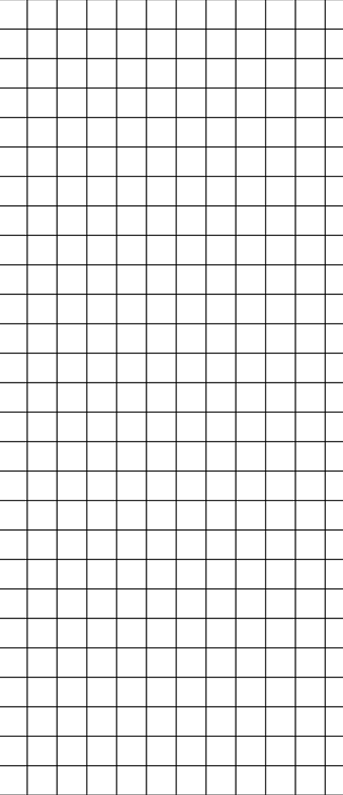Math Constructed Response: Show Me - Tell Me

 Show Me: What is the problem? Write what you know, make an Estimate, then use a Picture, Table, Graph with Labels, Patterns or Try-Check-Revise. Write an Equation to solve. Tell Me: How did you solve this problem? Why did you do it that way? Use math vocabulary. Use transition words (first, next, then, finally, etc.)